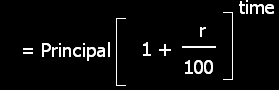One of my student has asked me to calculate number of years in compound interest. We know that compound interest is the interest on principle and total past interest. We can calculate compound interest by deducting principal from amount. It means, we have to calculate amount for calculating compound interest. But if amount is given, we can calculate number of years with following formula

But sometime, you can a complex problem in it. For example, student wants to know number of years when two type of principals are given. One's value will appreciate and other's value depreciate with same rate. Read this problem

A building worth Rs 1464100 is constructed on land worth Rs 1000000. After how many years will the value of both the same if land appreciates at 10% p.a. and building depreciates at 10% p.a.?
In this type of problem, we will put the value of principals of building and land but we will show time in building amount formulas as negative because when time will spend, our building value will decrease with same rate. Now, when we will show both formula after putting value, we can calculate the value of time. Following  tutorial will explain it in simple English.

Related : Interest Rate Risk.

## \$type=three\$a=hide\$cm=hide\$s=hide\$show=/2019/06/10-steps-to-become-better-investor.html\$l=hide

Name

false
ltr
item
Accounting Education: How to Calculate Number of Years in Compound interest
How to Calculate Number of Years in Compound interest